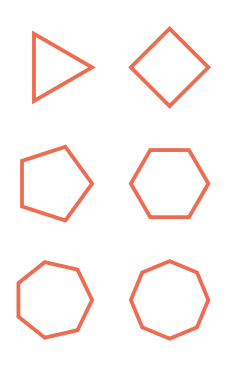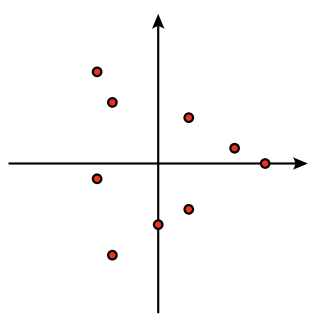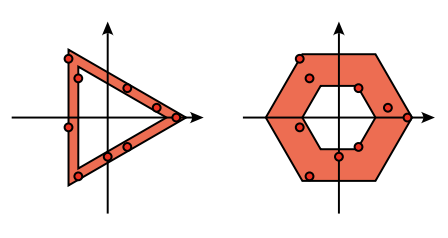시간 제한메모리 제한제출정답맞힌 사람정답 비율
2 초 512 MB33262578.125%

## 문제

You are an archaeologist working at an excavation site where your team has found hundreds of clay tablets containing glyphs written in some ancient language. Not much is known about the language yet, but you know that there are only six different glyphs, each of them in the shape of a regular polygon with one vertex pointing to the right (see Figure G.1(a) below). Only the boundary of each polygon is carved out of the clay.(a) The six glyphs. (b) The first sample input. (c) Fitting triangles and hexagons to the first sample. The triangles’ score is higher.

Figure G.1

You want to start analysing the language right away, so you need to get the text on the tablets into some machine readable format. Ideally, you would like to use an OCR (optical character recognition) tool for that, but you do not have one installed on your laptop and there is no internet connection at the site.

Because of this you have devised your own scheme to digitise the ancient writings: for every glyph on a tablet you first find a number of sample points that are in the carved out region, i.e. on the boundary of the polygon. Based on those sample points you then calculate a score for each of the six glyphs and mark the one with the highest score as the recognised glyph.

For a given number of corners k (3 ≤ k ≤ 8), the score is computed as follows. Two regular k-gons are fitted to the sample points, one from the inside and one from the outside, such that the following hold:

• Each polygon is centered at the origin, i.e. all vertices have equal distance to (0, 0).
• Each polygon has a vertex on the positive x-axis.
• The inner polygon is the largest such polygon containing none of the sample points.
• The outer polygon is the smallest such polygon containing all of the sample points.

An example can be seen in Figure G.1(c). The score for this value of k is Ainner/Aouter , where Ainner and Aouter are the areas of the inner and outer polygon, respectively.

Given a set of sample points, find the glyph with the highest score.

## 입력

The input consists of:

• One line with one integer n (1 ≤ n ≤ 1 000), the number of sample points.
• n lines, each with two integers x, y (−106 ≤ x, y ≤ 106), specifying a point at coordinates (x, y).

No sample point is at the origin and all points are distinct.

## 출력

Output the optimal number of corners k (3 ≤ k ≤ 8), followed by the score obtained for that value of k. Your answer will be accepted if the absolute error does not exceed 10−6. If several values of k result in a score that is within 10−6 of the optimal score, any one of them will be accepted.

## 예제 입력 1

9
-4 -1
-4 6
-3 -6
-3 4
0 -4
2 -3
2 3
5 1
7 0


## 예제 출력 1

3 0.5625000000


## 예제 입력 2

4
1 0
0 1
-1 0
0 -1


## 예제 출력 2

8 1.0000000000


## 출처• 문제를 만든 사람: Paul Wild